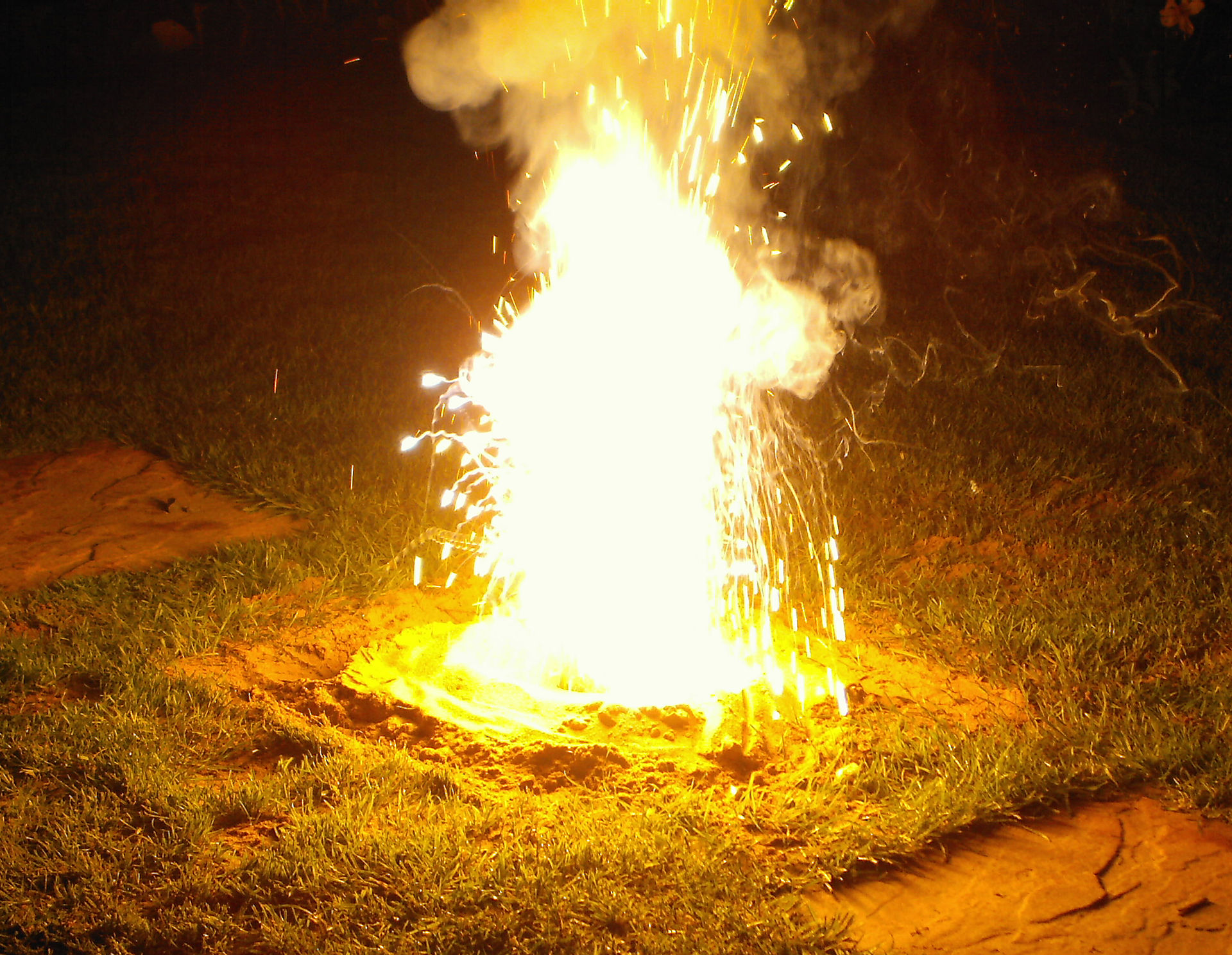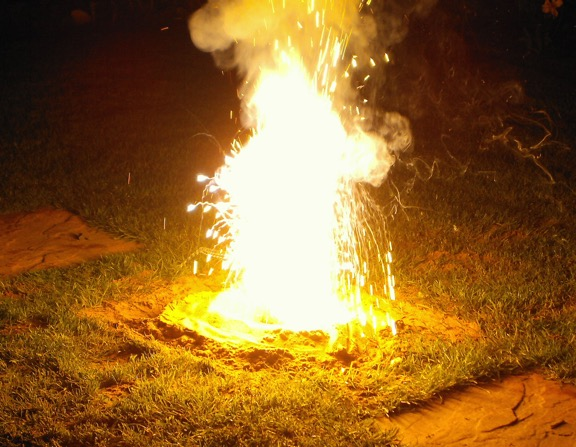## Blog Entries#### Oxidation-Reduction# RedOx reactions

The image above is of the Thermite reaction…from Wikipedia.
We have looked at acid/base and precipitation. The last class of reactions we will discuss is “oxidation/ reduction” reactions (also known as “RedOx" reactions). Precipitation is obvious, acid base is involves trading of protons (H+ ions). Oxidation/reduction involves trading electrons. For reasons that are sort of historical, oxidation is the losing of electrons, reduction is the gaining of electrons. You cannot have one without the other (the reason for the name is that oxygen is a very good oxidizer (though not the best). When oxygen interacts with other elements, it usually steals electrons. So, any time an element has electrons stolen from it, we say it was oxidized. The thing that steals the electrons is said to be “reduced.”

OIL RIG I’m not that fond of mnemonics. However, this one seems to help students. Oxidation Is Loss; Reduction Is Gain (of electrons).

These reactions are notable in a number of ways. I don't think it is too big of a stretch to say that they are the most important chemical reactions. Spontaneous RedOx reactions entail electrons going from a higher potential energy to a lower one, with a large release of Free Energy that can be used to do work. RedOx reactions include anything that is burning. Any combustion is a redox reaction.
Perhaps the most well-known oxidation/reduction reactions after burning things are those that are used in batteries. This makes sense. Remember that the defining characteristic of redox reactions is that there are electrons are traded. You might imagine that you could have the reactants in separated chambers with a wire to pass the electrons from one cell to the other. This would be a battery. In addition to batteries and
ALL combustion reactions (burning, as in all our fuel), all the the reactions in your body that allow you to use your food are redox reactions.

How do you recognize a redox reaction? Well, the only way is to look at the oxidation numbers of the various components. Two types we have looked at so far were “burning” oxidation (that is, really combining with oxygen) and cases where two metal ions “trade places.” Burning in oxygen is always an oxidation. The other class we looked at were metal exchanges. In these, one of the metals is in solution as a cation the other typically is a neutral metal. The thermite reaction where iron (iron III) end up stealing electrons from aluminum metal, releasing molten iron and aluminum oxide is one of the most spectacular redox reactions (see image above).
I have or will show you one in which Copper II in solution with sulfate stealing electrons from aluminum, leaving us with copper metal and Al
3+.

So, here are the definitions:
the thing that gets “reduced” ends up with a lower (reduced) oxidation number. The reduction is due to the gaining of electrons. Since it is reduced, something else must be oxidized by it. The thing that gains electrons stole them from some other ion or atom. It's oxidation number goes down (because it gains a negative thing—the electron(s)). So, it is reduced. Since it is acting like oxygen (steeling electrons) it is known as the "oxidizer" or "oxidizing agent." On the other hand, the thing that loses electrons gains in oxidation number. It is oxidized (as though it was beaten up by Oxygen). It is reducing the other thing, so it is the reducing agent.

## Assigning oxidation numbers:

Oxidation numbers really comprise nothing more than a book-keeping system for following electrons in a reaction. Unlike acid/base reactions, in which protons are traded (donated by the acid to the base) a “redox” reaction involves the trading of electrons. The thing that gains electrons gets “reduced,” and is the oxidizing agent (because it must oxidize something else to get those electrons). The thing that loses electrons gets “oxidized” and is the reducing agent. Reduction results in a lower oxidation number, because electrons are negatively charged. (have I said all that enough times yet?)
Okay, the way you know if a reaction is an oxidation/reduction (redox) reaction is simply to determine if the oxidation numbers of elements change. Remember that if one goes up, somebody else must go down. If an element loses electrons, some other element must gain them.
When dealing with mono-atomic ions, oxidation numbers and charges are the same thing. If sodium metal (which has no charge) reacts with chlorine gas (which also has no charge) the metal loses an electron to become Na+ while the chlorine gains one to become Cl-. The Cl is reduced (gains an electron, becomes lower, or negative, in charge) and is therefore the oxidizing agent--it oxidizes Na.
The Na is oxidized (loses electrons, becomes positive, or oxidation number goes up) and is the reducing agent (reduces chlorine).
The charge on the sodium is 1+ and it's oxidation state, or oxidation number…same thing…is +1 (yes, I meant to switch from 1+ for charge to +1 for oxidation state). Chloride has an oxidation state of -1.

## Covalent molecules

What about non-ionic interactions? Well, even in covalent interactions, the electrons are not shared equally. The electron goes from held by one element to held primarily by another. That's a lower PE level for the electron and therefore free energy is released.
Excluding the noble gases, which rarely partake in bonding, the biggest electron bullies are Fluorine and Oxygen. They will have the electrons more of the time, or, if you prefer the “electron cloud” analogy, has more of the cloud around it. So, for example, in water, the oxygen hogs the electrons donated by both of the hydrogens. Even though their are only partial charge, we pretend like the electron really is transferred from each hydrogen to the oxygen. We say that the hydrogens each have an oxidation number of +1 and that the oxygen therefore has an oxidation number of -2 (to account both of the two hydrogens). So, an Oxidation number is the charge that an element would have if the electrons involved in the bond were completely transferred to the more electronegative one. In the case of ionic compounds, they pretty much are. In covalent compounds, we just pretend they are for book-keeping practices.

#### The rules:

We have a property of elements we call "electronegativity." It's a measure of how tightly they hold electrons when bonded. The more electronegative elements will have negative oxidation numbers (they will hog the electrons) and the less electronegative elements will have positive oxidation numbers.
You can get the electronegativity from many periodic tables. The most electronegative thing is Fluorine and the least is Francium. The noble gases have no number for electronegativity.
Oxidation Numbers:
1. Atoms in their elemental state have an oxidation number of 0. This is true even in bonded elements, like diatomic gases or carbon in graphite or diamond. But, when in compounds:
2. Fluorine is always -1
3. Hydrogen is almost always +1
4. Oxygen is almost always -2. I’ll give you some hint if there is a peroxide, or -1 form, of oxygen present. If I don't specify it as a peroxide, you can assume that Oxygen is -2. The exceptions are metal hydride compounds which are important for batteries. We won't deal with them in this class much.
5. For metals, the oxidation number is the same as their charges: Alkali metals are always +1
6. Alkaline Earth metals are always +2
7. Other metals that have only one possible state are the same as for charge (silver, zinc and aluminum)

The sum of oxidation numbers must add to the total charge on the compound. That will be zero if it is a molecule. If it is a polyatomic ion, they will sum to the total charge on the ion. So, for SO
4 2-, The sum of the oxidation numbers must add up to -2. To get the states of the other elements in a compound, assign the easy ones first and then assign the other ones so that they add up. So, take SO4 2-. Each oxygen is -2 and there are 4 of them for a total of -8. The charge is 2- on the whole thing, so, Sulfur must be +6 ( +6 -8= -2)

### Common mistakes:

The most common mistake is assigning a non-zero oxidation number to an element. The numbers on the table tell you what the can be when they are in a compound. All elements (uncombined forms) have an oxidation number of 0 The second most common mistake is to confuse the individual oxidation state with the total for some identical atoms in a compound. For example, if you look at H
2O, you might say that the hydrogens are +2 to offset the -2 of the oxygen. But that is the total for ALL the hydrogens. The oxidation number on hydrogen is +1.

### Problems

Below there are two problems, one I did concerning calculating pH from concentrations and one looking at assigning oxidation numbers.
###### Acid/Base, pH.

45.0ml of 0.125M Magnesium chloride is added to 28.0 ml of 0.500M Sodium hydroxide.  Write the molecular, complete ionic and net ionic equations (noting spectator ions).  If a ppt is produced determine the theoretical yield.  Tell me what the pH of the initial  28.0 mls of base was before the magnesium chloride was added and tell me the final pH of the soln after the reactants were mixed.

Mg2+aq + 2Cl-(aq) + 2Na+(aq) + 2OH-(aq) Mg(OH)2(s) + 2Na+(aq) + 2Cl-(aq)

pOH= -log0.5= 0.301 pH=14-0.301= 13.7

Spectators are Na
+ and Cl-

0.028L x 0.5M = 0.014mol NaOH

0.125M x 0.045L = .00563 mol MgCl
2

Need 2NaOH/1MgCl
2 or, 0.01126 mol…have 0.014 mol. So, NaOH is in excess.

MgCl
2 is limiting. So, make 0.00563 mol of Mg(OH)2
0.00563mol x 58.32g/mol= 0.328g Mg(OH)
2

Consume 2x(0.00563) mol NaOH= 0.01126 (.0113)

mol OH
- left = 0.014-0.01125=.00275 mol

divide by new volume (0.073)
0.00275mol/0.073L= 3.77e-2 M

[OH
-] = 3.77e-2 pOH= 1.42

pH 12.6

###### Oxidation numbers:

Assign oxidation numbers to all the atoms in the barrium carbonate precipitation reaction: BaCl
2 (aq) + Na2CO3(aq) BaCO3(s)+ 2NaCl(aq)
Are any of the atoms changing oxidation state? Therefore, is this an oxidation/reduction reaction?
Next, Assign the oxidation numbers to all the atoms in this reaction: Fe
2O3(s) + 2Al(s) Al2O3(s) +Fe(l)
Is this an oxidation/reduction reaction? See you soon, Love, doc
Back
MathJax Font